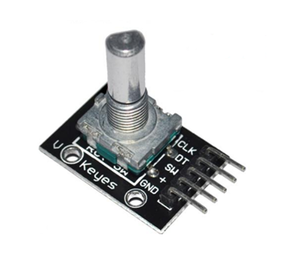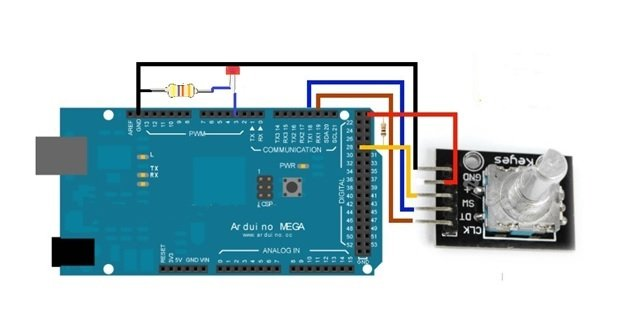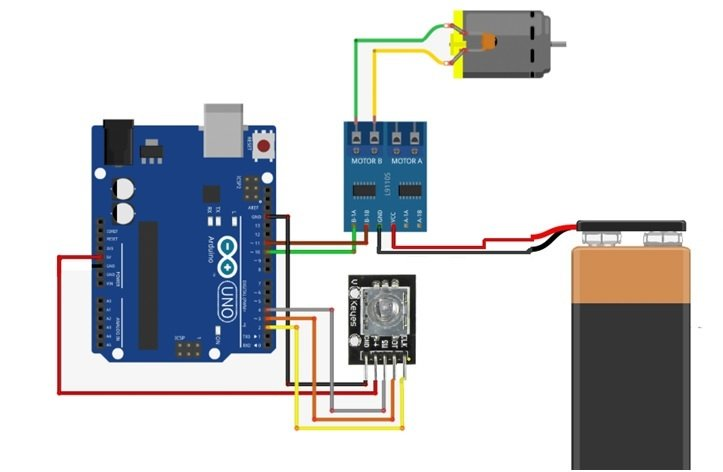# rotary encoder interfacing with arduino

Rotary Encoder: A rotary is a device that you can use to rotate infinitely. It may look to you like a potentiometer but it is different in operation. It is a type of position sensor which you can use to determine the angular position. It generates an analog or digital signal depending on the rotational movement. These can be used to control the brightness of led, controlling the servos and many other things.The rotary encoder has no starting, middle or end, so you can rotate it as much as you want. On many rotary encoders, when you will move it, you will feel a bump. These are the known as steps. Most have 12 steps, but they can be up to 200.There are many types of rotary encoder available which are either classified as the output signal or the sensing technology. The one we are using is the output rotary encoder and it is the simplest one.

### How Rotary Encoders Work?

The rotary encoder gives us two square waves output (A and b) which are 90 degrees out of phase which each other and the number of pulses completed each turn varies.We read the value of pulse B when every time A signal pulse goes from positive to zero. When the encoder is turned clockwise, B pulse is positive and when the encoder is turned counter-clockwise, the B pulse is negative. So, we can determine the direction of turn by checking both outputs with micro-controller and by counting the number of A pulses. We can also determine the speed by counting the frequency of pulses. So, you can see that it has a lot of advantages and it is much more applications than a simple potentiometer. In this tutorial we are going to use it in the simplest of applications. We will control the brightness of an led by using it.

### rotary encoder Pin Out

The rotary encoder has 5 pins which are as follows.• CLK: Encoder pin A
• DT: Encoder pin B
• SW: No push Button Switch
• +5V: 5V power Supply
• GND: Encoder Pin C

The module is designed such that the output will be low when the switches will be closed and it will be high when the switches will be open.

### Arduino interfacing with rotary encoderFirst of all connect the 5V and the Gnd of the encoder with the 5V and Gnd of the Arduino. Then connect the encoder pin A and Encoder pin B which are CLK and DT to the Arduino pin 19 and 18 respectively. Then connect the encoder push button pin (SW) to the Arduino pin 28. In last we have to connect the led whose brightness we will control. Connect the positive side of led to the pin 3 of Arduino and the negative side to the ground of Arduino. So, when we will move the rotary encoder in one direction, then the brightness of the led will increase and when we will use it in other direction then the brightness of the led will decrease. This is the simplest example in which we can use it. However, we can also control the motor and many other things using it.

### Code rotary encoder to control brightness of LED’s

This code is for the rotary encoder to control the brightness of led

```int brightness = 120 ;                                       // how much led will be bright at start

unsigned long currentTime ;

unsigned long loopTime ;

const int pin_A = 19;                                  // initializing pin 19 for encoder pin A

const int pin_B = 18;                               // initializing pin 18 for encoder pin B

int pushbutton = 28;                               // initializing pin 28 for push button.

unsigned char encoder_A;

unsigned char encoder_B;

unsigned char encoder_A_prev=0;

void setup ( )  {

pinMode ( 3 , OUTPUT ) ;                       // declaring pin 3 as output for the led

pinMode ( pin_A , INPUT ) ;                    // declaring pin 19 as input

pinMode ( pin_B , INPUT ) ;                   // declaring pin 18 as input

currentTime = millis ( ) ;

loopTime = currentTime ;

}

void loop ( )  {

currentTime = millis ( ) ;                           // This command will get the current elapsed time

if ( currentTime >= ( loopTime + 5 ) ){              // 5ms since last check of encoder = 200Hz

encoder_A = digitalRead ( pin_A ) ;                 // Reading the encoder pins

encoder_B = digitalRead ( pin_B ) ;                 // Reading the encoder pins

if ( ( !encoder_A ) && ( encoder_A_prev ) ) {

// A has gone from high to low

If ( encoder_B )  {                                  // encoder is moved clock wised so B is high

If ( brightness + fadeAmount <= 255 ) brightness += fadeAmount ;    // This command will increase the brightness

}

else {

// if encoder is moved counter-clockwise then b will be low

If ( brightness - fadeAmount >= 0 ) brightness -= fadeAmount ;       // This will decrease the brightness

}

}

encoder_A_prev = encoder_A ;                                                                            // this will Store value of A for next time

analogWrite ( 3, brightness ) ;                                                                                  // This will set the brightness of pin 3:

loopTime = currentTime ;

}

}```

### Controlling a Motor using Rotary EncoderIn this tutorial we are going to control a dc motor using the rotary encoder. To power a motor we will use a 9V power supply and a L91105 motor controller.

### Dc motor speed control with rotary encoder using Arduino

```volatile boolean Turnfound;                        // initializing a Boolean turn

volatile boolean up;                             // initializing a Boolean for speed up

const int CLK=2;   // initializng a const int for clock signal

const int DT=3;    // initializng a const int for Reading DT signal

const int SW=4;    // initializng a const int for Reading Push Button switch

// Connections for L9110 motor controller

#define L9110_B_IA 10                                        // initializing pins for motor

#define L9110_B_IB 11

// Motor Speed & Direction

#define MOTOR_SPEED L9110_B_IA                                       // Motor PWM Speed

#define MOTOR_DIRECTION L9110_B_IB                                              // Motor Direction
// Interrupt routine runs if CLK goes from HIGH to LOW

void isr ()  {

delay(4);                                                                                              // giving a delay

else

Turnfound = true;

}

void setup ()  {

pinMode(CLK,INPUT);                                // initializing clk pin as input

pinMode(DT,INPUT);                                 // initializing DT pin as input

pinMode(SW,INPUT);                                // initializing SW pin as input

digitalWrite(SW, HIGH);                           // Pull-Up resistor for switch

attachInterrupt (0,isr,FALLING);

Serial.begin (9600);                             // Setting baud rate at 9600

Serial.println("Start");

pinMode( MOTOR_DIRECTION, OUTPUT );

pinMode( MOTOR_SPEED, OUTPUT );

digitalWrite( MOTOR_DIRECTION, LOW );       // This will Set motor to off

digitalWrite( MOTOR_SPEED, LOW );

}

void loop ()  {

static long RotaryPosition=0;

if (RotaryPosition == 0)

} else {

RotaryPosition=0;

digitalWrite( MOTOR_DIRECTION, LOW );                        //This will turn motor off

analogWrite( MOTOR_SPEED, LOW );

Serial.print ("Reset = ");

Serial.println (RotaryPosition);

}

}

// Runs if rotation was detected

if (Turnfound)  {

if (up) {

if (RotaryPosition >= 100) { // Max value set to 100

RotaryPosition = 100;

}else {

RotaryPosition=RotaryPosition+2;}

}else {

if (RotaryPosition <= -100) { // Max value set to -100

RotaryPosition = -100;}

else {

RotaryPosition=RotaryPosition-2;}}

Turnfound = false;  // do NOT repeat IF loop until new rotation detected

Serial.print ("Speed = ");

Serial.println (RotaryPosition);        // if Rotation is Clockwise

if (RotaryPosition > 0 && RotaryPosition < 11) {

digitalWrite( MOTOR_DIRECTION, LOW );                                                      //This will turn off the motor

analogWrite( MOTOR_SPEED, LOW );

}

if (RotaryPosition > 10 && RotaryPosition < 21) {

digitalWrite( MOTOR_DIRECTION, HIGH );                                                       // direction will be forward

analogWrite( MOTOR_SPEED, 180 );                                   // PWM speed will be 180

}

if (RotaryPosition > 50 && RotaryPosition < 61) {

digitalWrite( MOTOR_DIRECTION, HIGH );                                                       // direction will be forward

analogWrite( MOTOR_SPEED, 100 );                                   // PWM speed will be 100

}

if (RotaryPosition > 90) {

digitalWrite( MOTOR_DIRECTION, HIGH );                                                       // direction will be forward

analogWrite( MOTOR_SPEED, 20 );                                                     // PWM speed will be 20

}

// if Rotation is Counter-Clockwise

if (RotaryPosition < 0 && RotaryPosition > -11) {

digitalWrite( MOTOR_DIRECTION, LOW );                                                      // This will turn off the motor

analogWrite( MOTOR_SPEED, LOW );

}

if (RotaryPosition < -10 && RotaryPosition > -21) {

digitalWrite( MOTOR_DIRECTION, LOW );                                                        // direction will be reverse

analogWrite( MOTOR_SPEED, 40 );                                                     // PWM speed will be 40

}

if (RotaryPosition < -40 && RotaryPosition > -51) {

digitalWrite( MOTOR_DIRECTION, LOW );                                                        // direction will be reverse

analogWrite( MOTOR_SPEED, 100 );                                   // PWM speed will be 100

}

if (RotaryPosition < -80 && RotaryPosition > -91) {

digitalWrite( MOTOR_DIRECTION, LOW );                                        // direction will be reverse

analogWrite( MOTOR_SPEED, 180 );                   // PWM speed will be 180

}

if (RotaryPosition < -90) {

digitalWrite( MOTOR_DIRECTION, LOW );                                        // direction will be reverse

analogWrite( MOTOR_SPEED, 200 );                   // PWM speed will be 200

}

}}```

### 3 thoughts on “rotary encoder interfacing with arduino”

1. Hi Bilal Malik

2. Motor-DC-Encoder-Control-Pr01.ino: In function ‘void loop()’:
Motor-DC-Encoder-Control-Pr01.ino:69:22: error: ‘If’ was not declared in this scope
Motor-DC-Encoder-Control-Pr01.ino:69:25: error: expected ‘;’ before ‘{‘ token
Motor-DC-Encoder-Control-Pr01.ino:75:7: error: expected ‘}’ before ‘else’
Motor-DC-Encoder-Control-Pr01.ino:79:43: error: ‘If’ was not declared in this scope
Motor-DC-Encoder-Control-Pr01.ino:79:45: error: expected ‘;’ before ‘brightness’
Motor-DC-Encoder-Control-Pr01.ino: At global scope:
Motor-DC-Encoder-Control-Pr01.ino:93:1: error: expected declaration before ‘}’ token
Fehler beim Kompilieren.

• // this is the correct code in the void loop. kindly replace

void loop () {

static long RotaryPosition=0;

if (RotaryPosition == 0){

} else {
{

RotaryPosition=0;

digitalWrite( MOTOR_DIRECTION, LOW ); //This will turn motor off

analogWrite( MOTOR_SPEED, LOW );

Serial.print (“Reset = “);

Serial.println (RotaryPosition);

}

}